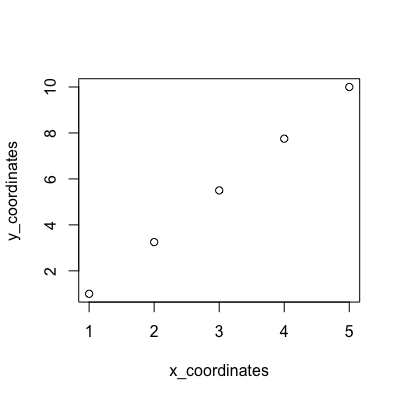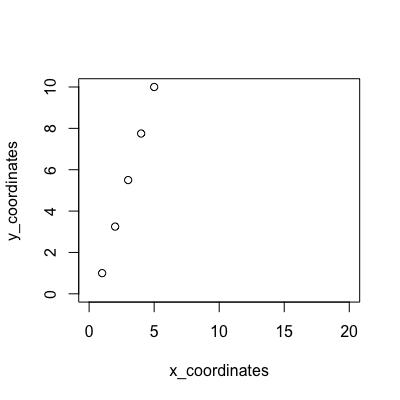Open in App
Not now

# How to set x and y limits for qqplot using car package in R

• Last Updated : 21 Nov, 2022

In this article, we are going to know how to set x and y limits for qqplot using car package in the R programming language.

The “car” package in R, which defines Companion to Applied Regression is used to perform regressive tests as well as data visualizations. It is also used to carry out data transformations. The package can be installed into the working space using the following command :

`install.packages("car")`

A vector of x and y coordinates can be specified to define the points in the R working space. The qqplot() method in the “car” package can then be invoked to construct a graph of the coordinates. The quantile-quantile plot, also referred to as the probability plot can be constructed using this package. It specified the x and y coordinates of the points to be plotted.

Syntax: qqplot(x , y)

Parameters:

• x – numeric vector of observations of x.
• y – numeric vector of observations of y.

## Example 1:

In this example, we are plotting the quartile-quartile graph using qqplot() function. firstly we are storing the x and y coordinates using a vector and then printing the graph using qqplot(). The qqplot() method access the coordinates by default based on the values of the data points and make the x and y axis scale according to them.

## R

 `# Importing library "car"``library``(``"car"``)`` ` `# Declaring the x and y coordinates ``x_coordinates <- ``c``(1:5)``y_coordinates <- ``c``(1:10)`` ` `# Default x-axis from 1 to 5 and ``# y-axis from 1 to 10``qqplot``(x_coordinates, y_coordinates) `

Output:## Example 2:

In this example, we are plotting the Q-Q plot as in the first example but extra work going to do in this example is to make a customized scale, and set the x and y axes limits respectively, and the xlim and ylim parameters in qqplot() method can be added. The xlim and ylim arguments take a vector containing the start and end of the respective x and y axes to be plotted.

## R

 `# Importing library "car"``library``(``"car"``)`` ` `# Declaring the x and y coordinates ``x_coordinates <- ``c``(1:5)``y_coordinates <- ``c``(1:10)`` ` ` ` `# Set limit of x-axis from 0 to 10 ``# and y-axis from 0 to 20``qqplot``(x_coordinates, y_coordinates, ``       ``ylim = ``c``(0, 10), ``       ``xlim = ``c``(0, 20))`

Output:My Personal Notes arrow_drop_up